# CVPR2022 | Accuracy和F1-score能代表车道线检测网络性能吗？ 原创

0收藏

## 摘要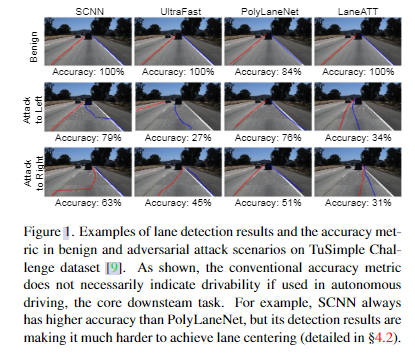## 相关工作

### 车道线检测的评价指标

1. 没有理由设置 α = 20 像素和 β = 0.85 为精度阈值。例如，即使检测误差超过20个像素，只要检测到的车道线与实际车道线平行，ALC系统也可以保持在车道中心。
2. 此外，检测到的车道线点的重要性不应该相等，即离车辆较近的点应该比距离较远的点更重要以控制车辆。当前指标平等对待驾驶员视野中的所有车道线，例如，当前车道左侧线的检测错误与左侧车道左侧线的检测错误被视为相同重要性。然而，前者对 ALC 系统来说比后者重要得多，因为前者可以直接影响车道中心的下游计算。例如，如果模型无法检测到左侧车道的左侧线，但仍然可以检测到当前车道的左侧线，则不会影响其用于 ALC。

## 方法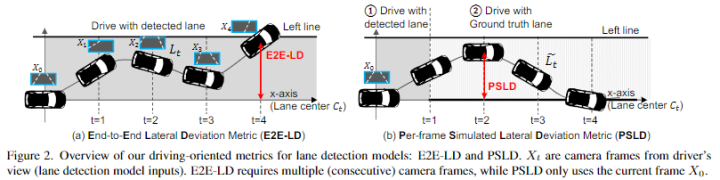### 端到端横向偏差度量（End-to-End Lateral Deviation Metric）（E2E-LD）

ALC的性能通过车辆在车道中心行驶的准确程度来评估，即与车道中心的横向（左或右）偏差。 连续闭环感知和控制中车辆与车道中心的最大横向偏差是车道检测的最终下游任务性能指标。 这种偏离是直接影响安全的，因为大的横向偏离可能导致与其他驾驶车辆或路边物体的致命碰撞。 我们称之为端到端横向偏差度量（E2E-LD），如图 2（a）所示。$t = 0$ 时的 E2E-LD 如下获得。

$$\mathop{max}\limits_{t \leq T_E}(|L_t-C_t|)$$

$L_t$ 是车辆在 t 的横向（y 轴）坐标。$C_t$是对应于 t 处车辆位置的车道中心横向（y 轴）坐标。我们使用在$t=0$时的车辆坐标系。$T_E$ 是决定持续时间的超参数。如果$T_E=1s$ ，E2E-LD是一秒内的最大偏差。要获得$L_t$ ，需要一个闭环机制来模拟 ALC 的运行，例如 AD 模拟器 [3, 25]。从$t = 0$开始，$t = 1$时的车辆位置和航向基于$t = 0$时的图像帧（camera frames）（$X_0$）计算：车道检测模型从图像帧中检测车道线，进行横向控制，操作方向盘。重复此过程，直到$t = T_e$。 因此，需要多个（连续的）图像帧（camera frames）$X_0，…，X_{T_E}$，而且它们会随着较早帧中的车道检测结果的变化而动态更改。

### 每帧模拟横向偏差度量（Per-Frame Simulated Lateral Deviation Metric）(PSLD)

E2E-LD metric 定义为基于下游任务 ALC 要求的期望的 metric。 然而，在上游车道检测模型的训练过程中，它仍然需要大量的计算来监控。 这种开销主要是由于图像帧（camera frames）的相互依赖性，即图像帧会根据较早帧中的车道检测结果动态更改。 为了解决这个限制，我们设计了每帧模拟横向偏差指标 (PSLD)，它仅使用当前帧 ($X_0$) 的单个图像输入和车道中心的几何形状来模拟 E2E-LD。

PSLD 的概述如图 2（b）所示。计算包括两个阶段：

1. 使用 $t = 0$ ($X_0$) 处的当前图像帧及其车道检测结果更新车辆位置
2. 使用真值车道中心作为 waypoints 从$t = 1$应用闭环模拟 至 $t = T_p$。

$$\frac{1}{T_{p}} \max {1 \leq t \leq T{p}}\left(\left|\widetilde{L}{t}-C{t}\right|\right)$$

### 攻击产生

$$\frac{1}{L \cdot H} \sum_{l=1}^{L} \sum_{i=1}^{W} \sum_{j=1}^{I I} i \cdot P_{i j}^{l}$$

$$\frac{1}{L \cdot|\mathcal{H}|} \sum_{l=1}^{L} \sum_{j \in \mathcal{H}}\left[j^{d}, j^{d-1}, \cdots, j, 1\right] p_{l}$$

$L$ 是检测到的车道线的数目，$d$ 是多项式的次数，$\mathcal{H}$ 是y轴采样行数值的集合，$p_{l} \in \mathbb{R}^{d+1}$ 是检测到的车道线$l$的系数。

$$\sum_{l \in \mathcal{A}}\left[\frac{1}{\left|\Delta^{l}\right|} \sum_{j \in \Delta^{l}}\left(a_{j}^{l}+\delta_{j}^{l}\right)\right] \cdot \pi^{l}$$

## 实验

### TuSimple数据集的常规评估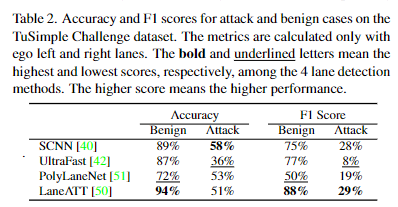### TuSimple 指标与 E2E-LD 的一致性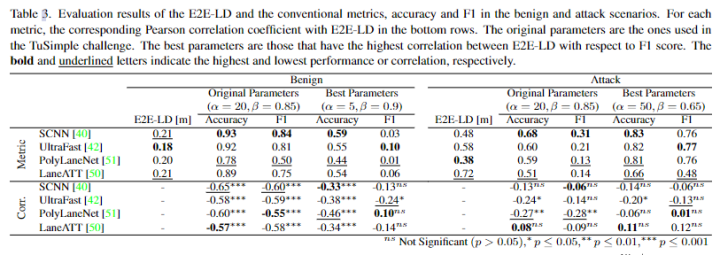E2E-LD 与传统指标之间的不一致可以使用 Pearson 相关系数 r 更系统地量化。 一般来说，E2E-LD 和传统指标具有很强的负相关性 (r ≤-0.55)，具有很高的统计显着性 (p ≤0.001)，这意味着最近对传统指标的一些改进可能并没有导致 AD 的改进，相反，过度拟合传统指标可能会使情况变得更糟。

### E2E-LD与PSLD的一致性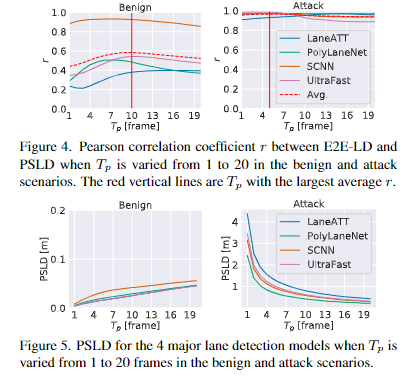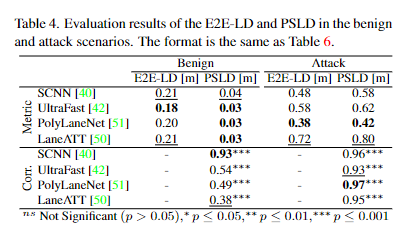## 参考

 Towards Driving-Oriented Metric for Lane Detection Models